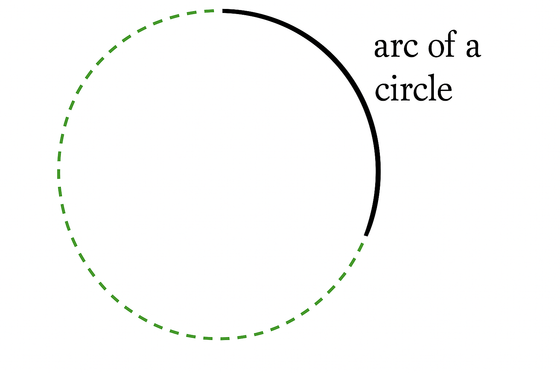# arc of a circle

Arc of a Circle

A connected section of the circumference of a circle.Arcs are measured in two ways: as the measure of the central angle, or as the length of the arc itself.

 Measurement by central angle (degrees)The red arc (minor arc) measures 120°.The blue arc (major arc) measures 240°. Measurement by arc length (radians)Formula: s = rθs = arc lengthr = radius of the circleθ = measure of the central angle in radiansRed arc:  r = 2 and θ = 2π/3, so s = 4π/3Blue arc:  r = 2 and θ = 4π/3, so s = 8π/3